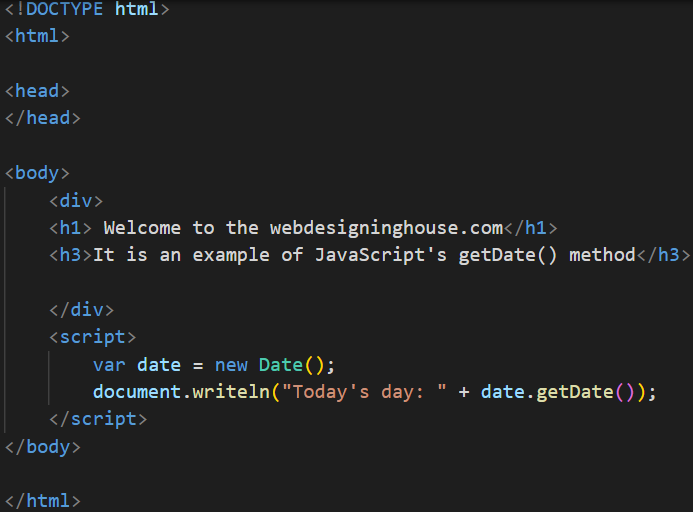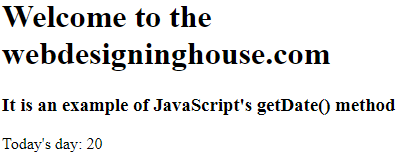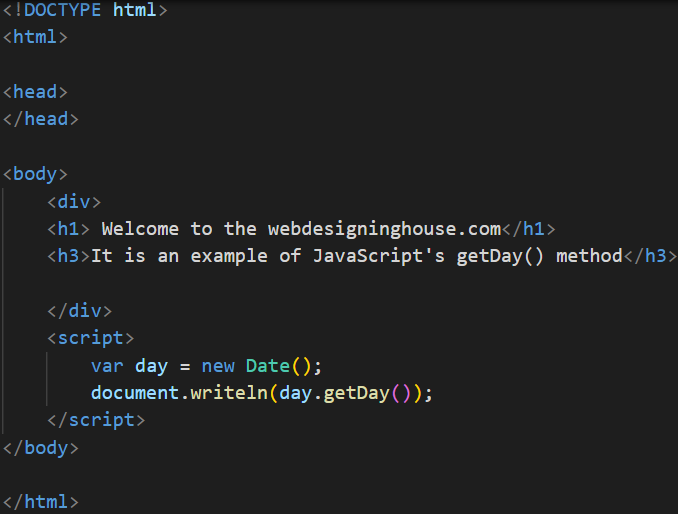JavaScript Get Date Methods

# JavaScript Get Date Methods

JavaScript Get Date Method
Commonly used Date and Time Methods are as follows:
 Name Description getDate() Get the day of the month as an integer from 1 to 31 getDay() Get the day of the week as an integer where 0 is Sunday and 1 is Monday getHours() Get the hours as an integer between 0 and 23 getMinutes() Get the minutes is an integer between 0 and 59 getMonth() Get the Months as an integer between 0 and 11 where 0 is January and 11 is December getSeconds() Get the seconds as an integer between 0 and 59 getTime() Get the current time in milliseconds where is milliseconds since January 1, 1970 getYear() Get the year, but this format differs from browser to browser
Example1 – Example of JavaScript ‘s  getDate() method:OutputExample2Output